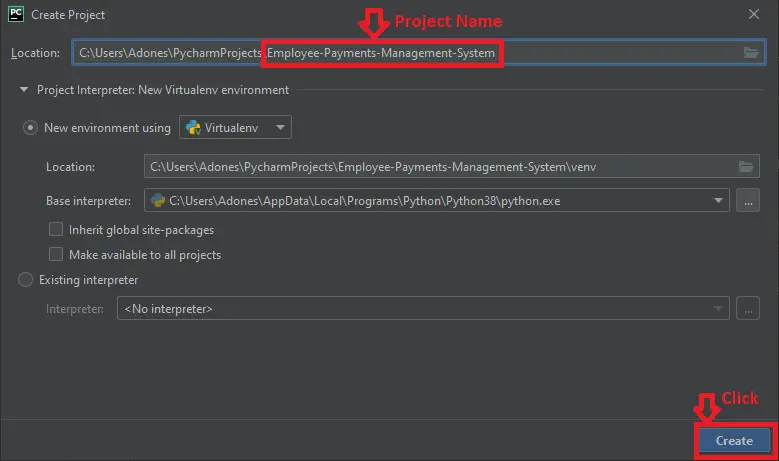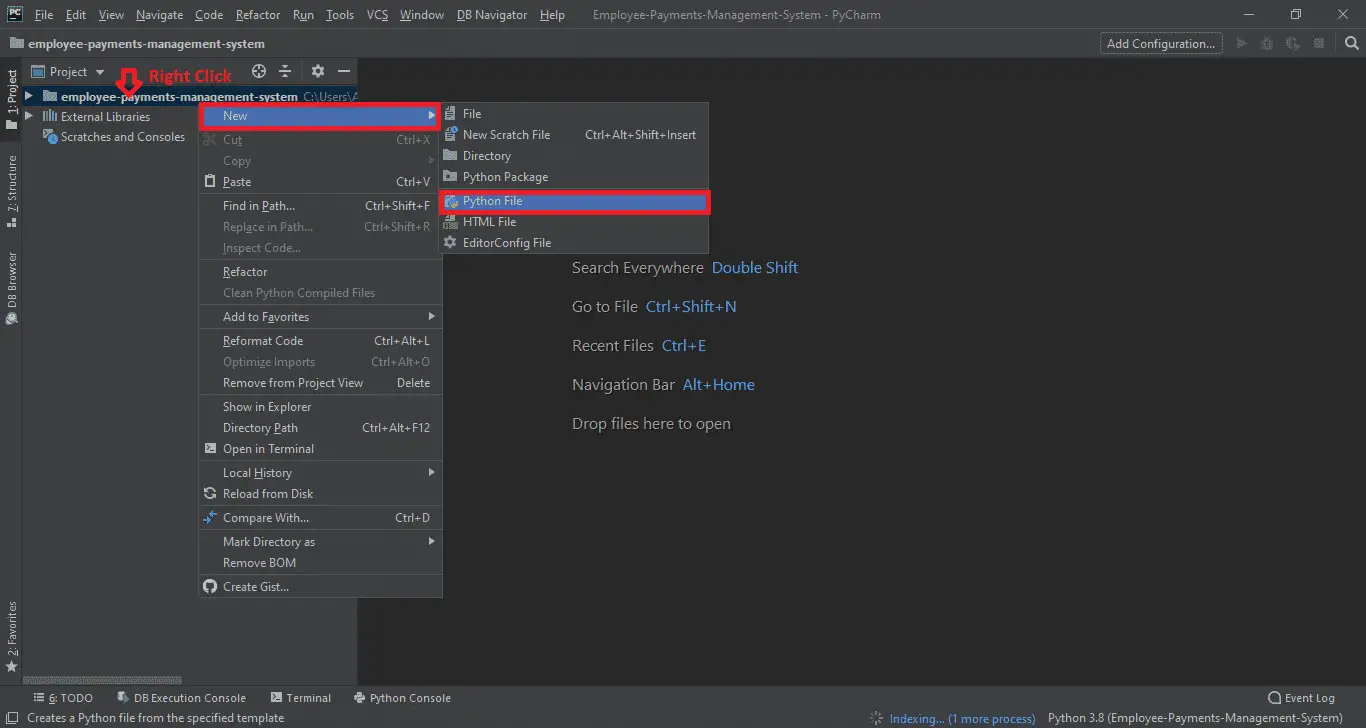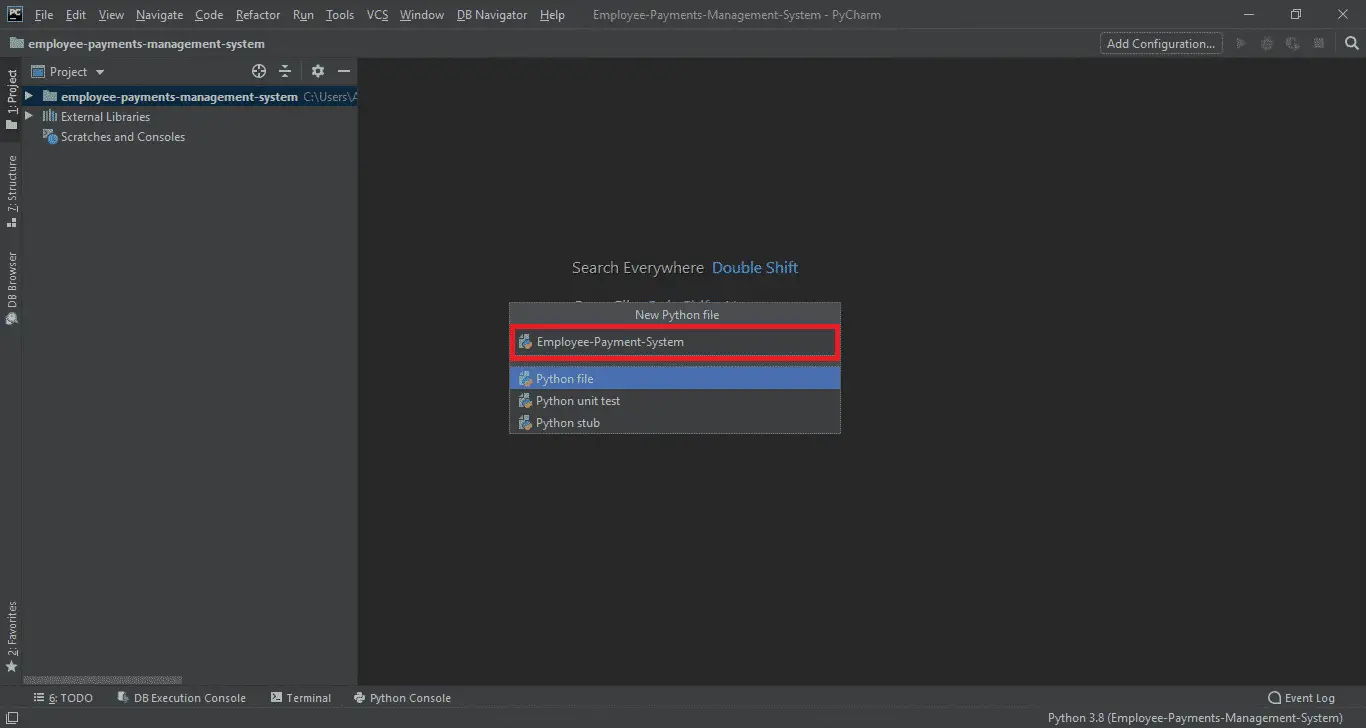# Employee Payment Management System Project in Python

## Employee Payment Management System Project in Python

This Employee Payment Management System Project in Python is essentially a desktop software developed using Pycharm and Tkinter platform. Patient Information consists of a Python Script (Employee-Payment-System.Py). Instead, For teaching purposes that is an smooth and essential degree tiny project. This Python challenge with academic and manual for growing a code.

Employee Payment Management System is a open supply you can Download zip and edit as according to you need. If you need more present day Python tasks here. This is simple and simple stage small undertaking for studying purpose.

Also you may modified this device as consistent with your requirements and develop an excellent advance stage mission. Zip report containing the supply code that may be extracted after which imported into pycharm.

To create a Employee Payment Management System Project in Python. Make certain you have got PyCharm IDE installed for your computer.

By the way if you are new to python programming and you don’t know what would be the the Python IDE to use, I have here a list of Best Python IDE for Windows, Linux, Mac OS that will suit for you. I also have here How to Download and Install Latest Version of Python on Windows.

## This are the steps on how to create  Employee Payment Management System Project in Python.

Employee Payment Management System Project in Python

• Step 1: Create a Project Name

First step open the PyCharm IDE and click “File” and select “New Project” and then create a project name after that click the “create” button.• Step 2: Create a Python File

Second step after creating a project name, “right” click the project name and the click “New” after that choose “Python File“.• Step 3: Name the Python File

Third step after choose Python File name the file “Employee-Payment-System” and then click “Enter“.• Creating a File name in Employee Payment Management System

Now you can start coding, you are free to copy the code that being provided below.

### This Code Below, Which is for the Whole Frame Window of Employee Payment Management System.

```root = Tk()
root.title("Employee Payment System")
root.geometry('1370x720+0+0')
root.maxsize(width=1370, height=720)
root.minsize(width=1370, height=720)
root.configure(background="dark gray")

Tops = Frame(root, width=1350, height=50, bd=8, bg="dark blue")
Tops.pack(side=TOP)

f1 = Frame(root, width=600, height=600, bd=8, bg="dark gray")
f1.pack(side=LEFT)
f2 = Frame(root, width=300, height=700, bd=8, bg="dark blue")
f2.pack(side=RIGHT)

fla = Frame(f1, width=600, height=200, bd=8, bg="dark blue")
fla.pack(side=TOP)
flb = Frame(f1, width=300, height=600, bd=8, bg="dark blue")
flb.pack(side=TOP)

lbl_information = Label(Tops, font=('arial', 45, 'bold'), text="Employee Payment Management System ", relief=GROOVE, bd=10, bg="Dark Gray", fg="Black")
lbl_information.grid(row=0, column=0)```

### This module is for exit function of employee payment management system

```def Exit():
wayOut = tkinter.messagebox.askyesno("Employee Payment System", "Do you want to exit the system")
if wayOut > 0:
root.destroy()
return```

### This module is for the reset function of employee payment management system

```def Reset():
FullName.set("")
Wrk_Hrs.set("")
Hrs_Wage.set("")
Payable.set("")
Taxable.set("")
NetPayable.set("")
GrossPayable.set("")
OverTimeBonus.set("")
CompanyAgency.set("")
PhoneNumber.set("")
txtPaymentSlip.delete("1.0", END)```

### This function is for the view payslip of employee payment management system

```def InformationEntry():
txtPaymentSlip.delete("1.0", END)
txtPaymentSlip.insert(END, "\t\tPay Slip\n\n")
txtPaymentSlip.insert(END, "Full Name :\t\t" + FullName.get() + "\n\n")
txtPaymentSlip.insert(END, "Company/Agency :\t\t" + CompanyAgency.get() + "\n\n")
txtPaymentSlip.insert(END, "Phone Number :\t\t" + PhoneNumber.get() + "\n\n")
txtPaymentSlip.insert(END, "Hours Worked :\t\t" + Wrk_Hrs.get() + "\n\n")
txtPaymentSlip.insert(END, "Net Payable :\t\t" + NetPayable.get() + "\n\n")
txtPaymentSlip.insert(END, "Wages per hour :\t\t" + Hrs_Wage.get() + "\n\n")
txtPaymentSlip.insert(END, "Tax Paid :\t\t" + Taxable.get() + "\n\n")
txtPaymentSlip.insert(END, "Payable :\t\t" + Payable.get() + "\n\n")```

### This function is for the calculation payments of weekly wages of employee payment management system

```def WagesForWeekly():
txtPaymentSlip.delete("1.0", END)
hrs_wrk_per_wek = float(Wrk_Hrs.get())
hrs_per_wgs = float(Hrs_Wage.get())

DuePayment = hrs_per_wgs * hrs_wrk_per_wek
PaymentDue = "P" + str('%.2f' % DuePayment)
Payable.set(PaymentDue)

tax = DuePayment * 0.12
taxable = "P" + str('%.2f' % tax)
Taxable.set(taxable)

PaymentNet = DuePayment - tax
NetPayments = "P" + str('%.2f' % PaymentNet)
NetPayable.set(NetPayments)

if hrs_wrk_per_wek > 40:
HoursTimeOver = (hrs_wrk_per_wek - 40) + hrs_per_wgs * 1.5
OverTime = "P" + str('%.2f' % HoursTimeOver)
OverTimeBonus.set(OverTime)
elif hrs_wrk_per_wek <= 40:
PaymentOverTime = (hrs_wrk_per_wek - 40) + hrs_per_wgs * 1.5
HoursOverTime = "P" + str('%.2f' % PaymentOverTime)
OverTimeBonus.set(HoursOverTime)
return```

### This module below, Which is for the declaring variables function Use in employee payment management system

```FullName = StringVar()
Hrs_Wage = StringVar()
Wrk_Hrs = StringVar()
Payable = StringVar()
Taxable = StringVar()
NetPayable = StringVar()
GrossPayable = StringVar()
OverTimeBonus = StringVar()
CompanyAgency = StringVar()
PhoneNumber = StringVar()
TimeOfOrder = StringVar()
DateOfOrder = StringVar()
DateOfOrder.set(time.strftime("%d/%m/%Y"))```

### This code below, Which is for the label widget design use in employee payment management system

```lblFirstName = Label(fla, text="Full Name", font=('arial', 16, 'bold'), bd=20, fg="white", bg="dark blue").grid(row=0, column=0)

lblAddress = Label(fla, text="Home Address", font=('arial', 16, 'bold'), bd=20, fg="white", bg="dark blue").grid(row=0, column=2)

lblCompanyAgency = Label(fla, text="Company/Agency", font=('arial', 16, 'bold'), bd=20, fg="white", bg="dark blue").grid(row=1,
column=0)
lblPhoneNumber = Label(fla, text="Phone Number", font=('arial', 16, 'bold'), bd=20, fg="white", bg="dark blue").grid(row=1,
column=2)
lblHoursWorked = Label(fla, text="Hours Worked", font=('arial', 16, 'bold'), bd=20, fg="white", bg="dark blue").grid(
row=2, column=0)
lblHourlyRate = Label(fla, text="Hourly Rate", font=('arial', 16, 'bold'), bd=20, fg="white", bg="dark blue").grid(
row=2, column=2)
lblTax = Label(fla, text="Tax", font=('arial', 16, 'bold'), bd=20, anchor='w', fg="white", bg="dark blue").grid(row=3,
column=0)
lblOverTime = Label(fla, text="OverTime", font=('arial', 16, 'bold'), bd=20, fg="white", bg="dark blue").grid(row=3,
column=2)
lblGrossPay = Label(fla, text="GrossPay", font=('arial', 16, 'bold'), bd=20, fg="white", bg="dark blue").grid(row=4,
column=0)
lblNetPay = Label(fla, text="Net Pay", font=('arial', 16, 'bold'), bd=20, fg="white", bg="dark blue").grid(row=4,
column=2)```

### This module below, Which is for the text entry widget design use in employee payment management system

```txtFullname = Entry(fla, textvariable=FullName, font=('arial', 16, 'bold'), bd=16, width=22, justify='left')
txtFullname.grid(row=0, column=1)

txtCompanyAgency = Entry(fla, textvariable=CompanyAgency, font=('arial', 16, 'bold'), bd=16, width=22, justify='left')
txtCompanyAgency.grid(row=1, column=1)

txtWrk_hrs = Entry(fla, textvariable=Wrk_Hrs, font=('arial', 16, 'bold'), bd=16, width=22, justify='left')
txtWrk_hrs.grid(row=2, column=1)

txtHrs_Wages = Entry(fla, textvariable=Hrs_Wage, font=('arial', 16, 'bold'), bd=16, width=22, justify='left')
txtHrs_Wages.grid(row=2, column=3)

txtPhoneNumber = Entry(fla, textvariable=PhoneNumber, font=('arial', 16, 'bold'), bd=16, width=22, justify='left')
txtPhoneNumber.grid(row=1, column=3)

txtGrossPayment = Entry(fla, textvariable=Payable, font=('arial', 16, 'bold'), bd=16, width=22, justify='left')
txtGrossPayment.grid(row=4, column=1)

txtNetPayable = Entry(fla, textvariable=NetPayable, font=('arial', 16, 'bold'), bd=16, width=22, justify='left')
txtNetPayable.grid(row=4, column=3)

txtTaxable = Entry(fla, textvariable=Taxable, font=('arial', 16, 'bold'), bd=16, width=22, justify='left')
txtTaxable.grid(row=3, column=1)

txtOverTimeBonus = Entry(fla, textvariable=OverTimeBonus, font=('arial', 16, 'bold'), bd=16, width=22, justify='left')
txtOverTimeBonus.grid(row=3, column=3)

payslip = Label(f2, textvariable=DateOfOrder, font=('arial', 21, 'bold'), fg="white", bg="dark blue").grid(row=0,
column=0)
txtPaymentSlip = Text(f2, height=22, width=34, bd=16, font=('arial', 13, 'bold'), fg="black", bg="white")
txtPaymentSlip.grid(row=1, column=0)```

### This code below, Which is for the buttons of salary, reset, view payslip and exit use in employee payment management system

```ButtonSalary = Button(flb, text='Weekly Salary', padx=16, pady=16, bd=15, font=('arial', 16, 'bold'), relief="groove", width=14, fg="black",
bg="dark gray", command=WagesForWeekly).grid(row=0, column=0)

ButtonReset = Button(flb, text='Reset', padx=16, pady=16, bd=15, font=('arial', 16, 'bold'), relief="groove", width=14, command=Reset,
fg="black", bg="dark gray").grid(row=0, column=1)

ButtonPaySlip = Button(flb, text='View Payslip', padx=16, pady=16, bd=15, font=('arial', 16, 'bold'), relief="groove", width=14,
command=InformationEntry, fg="black", bg="dark gray").grid(row=0, column=2)

ButtonExit = Button(flb, text='Exit System', padx=16, pady=16, bd=15, font=('arial', 16, 'bold'), relief="groove", width=14, command=Exit,
fg="black", bg="dark gray").grid(row=0, column=3)```

### This module below, Which for the Main Module of the employee payment management system

```import time
import tkinter.messagebox
from tkinter import *

root = Tk()
root.title("Employee Payment System")
root.geometry('1370x720+0+0')
root.maxsize(width=1370, height=720)
root.minsize(width=1370, height=720)
root.configure(background="dark gray")

Tops = Frame(root, width=1350, height=50, bd=8, bg="dark blue")
Tops.pack(side=TOP)

f1 = Frame(root, width=600, height=600, bd=8, bg="dark gray")
f1.pack(side=LEFT)
f2 = Frame(root, width=300, height=700, bd=8, bg="dark blue")
f2.pack(side=RIGHT)

fla = Frame(f1, width=600, height=200, bd=8, bg="dark blue")
fla.pack(side=TOP)
flb = Frame(f1, width=300, height=600, bd=8, bg="dark blue")
flb.pack(side=TOP)

lbl_information = Label(Tops, font=('arial', 45, 'bold'), text="Employee Payment Management System ", relief=GROOVE, bd=10, bg="Dark Gray", fg="Black")
lbl_information.grid(row=0, column=0)

def Exit():
wayOut = tkinter.messagebox.askyesno("Employee Payment System", "Do you want to exit the system")
if wayOut > 0:
root.destroy()
return

def Reset():
FullName.set("")
Wrk_Hrs.set("")
Hrs_Wage.set("")
Payable.set("")
Taxable.set("")
NetPayable.set("")
GrossPayable.set("")
OverTimeBonus.set("")
CompanyAgency.set("")
PhoneNumber.set("")
txtPaymentSlip.delete("1.0", END)

def InformationEntry():
txtPaymentSlip.delete("1.0", END)
txtPaymentSlip.insert(END, "\t\tPay Slip\n\n")
txtPaymentSlip.insert(END, "Full Name :\t\t" + FullName.get() + "\n\n")
txtPaymentSlip.insert(END, "Company/Agency :\t\t" + CompanyAgency.get() + "\n\n")
txtPaymentSlip.insert(END, "Phone Number :\t\t" + PhoneNumber.get() + "\n\n")
txtPaymentSlip.insert(END, "Hours Worked :\t\t" + Wrk_Hrs.get() + "\n\n")
txtPaymentSlip.insert(END, "Net Payable :\t\t" + NetPayable.get() + "\n\n")
txtPaymentSlip.insert(END, "Wages per hour :\t\t" + Hrs_Wage.get() + "\n\n")
txtPaymentSlip.insert(END, "Tax Paid :\t\t" + Taxable.get() + "\n\n")
txtPaymentSlip.insert(END, "Payable :\t\t" + Payable.get() + "\n\n")

def WagesForWeekly():
txtPaymentSlip.delete("1.0", END)
hrs_wrk_per_wek = float(Wrk_Hrs.get())
hrs_per_wgs = float(Hrs_Wage.get())

DuePayment = hrs_per_wgs * hrs_wrk_per_wek
PaymentDue = "P" + str('%.2f' % DuePayment)
Payable.set(PaymentDue)

tax = DuePayment * 0.12
taxable = "P" + str('%.2f' % tax)
Taxable.set(taxable)

PaymentNet = DuePayment - tax
NetPayments = "P" + str('%.2f' % PaymentNet)
NetPayable.set(NetPayments)

if hrs_wrk_per_wek > 40:
HoursTimeOver = (hrs_wrk_per_wek - 40) + hrs_per_wgs * 1.5
OverTime = "P" + str('%.2f' % HoursTimeOver)
OverTimeBonus.set(OverTime)
elif hrs_wrk_per_wek <= 40:
PaymentOverTime = (hrs_wrk_per_wek - 40) + hrs_per_wgs * 1.5
HoursOverTime = "P" + str('%.2f' % PaymentOverTime)
OverTimeBonus.set(HoursOverTime)
return

# Variables
FullName = StringVar()
Hrs_Wage = StringVar()
Wrk_Hrs = StringVar()
Payable = StringVar()
Taxable = StringVar()
NetPayable = StringVar()
GrossPayable = StringVar()
OverTimeBonus = StringVar()
CompanyAgency = StringVar()
PhoneNumber = StringVar()
TimeOfOrder = StringVar()
DateOfOrder = StringVar()
DateOfOrder.set(time.strftime("%d/%m/%Y"))

# Label Widget

labelFirstName = Label(fla, text="Full Name", font=('arial', 16, 'bold'), bd=20, fg="white", bg="dark blue").grid(row=0, column=0)

labelAddress = Label(fla, text="Home Address", font=('arial', 16, 'bold'), bd=20, fg="white", bg="dark blue").grid(row=0, column=2)

labelCompanyAgency = Label(fla, text="Company/Agency", font=('arial', 16, 'bold'), bd=20, fg="white", bg="dark blue").grid(row=1,
column=0)
labelPhoneNumber = Label(fla, text="Phone Number", font=('arial', 16, 'bold'), bd=20, fg="white", bg="dark blue").grid(row=1,
column=2)
labelHoursWorked = Label(fla, text="Hours Worked", font=('arial', 16, 'bold'), bd=20, fg="white", bg="dark blue").grid(
row=2, column=0)
labelHourlyRate = Label(fla, text="Hourly Rate", font=('arial', 16, 'bold'), bd=20, fg="white", bg="dark blue").grid(
row=2, column=2)
labelTax = Label(fla, text="Tax", font=('arial', 16, 'bold'), bd=20, anchor='w', fg="white", bg="dark blue").grid(row=3,
column=0)
labelOverTime = Label(fla, text="OverTime", font=('arial', 16, 'bold'), bd=20, fg="white", bg="dark blue").grid(row=3,
column=2)
labelGrossPay = Label(fla, text="GrossPay", font=('arial', 16, 'bold'), bd=20, fg="white", bg="dark blue").grid(row=4,
column=0)
labelNetPay = Label(fla, text="Net Pay", font=('arial', 16, 'bold'), bd=20, fg="white", bg="dark blue").grid(row=4,
column=2)

# Entry Widget

txtFullname = Entry(fla, textvariable=FullName, font=('arial', 16, 'bold'), bd=16, width=22, justify='left')
txtFullname.grid(row=0, column=1)

txtCompanyAgency = Entry(fla, textvariable=CompanyAgency, font=('arial', 16, 'bold'), bd=16, width=22, justify='left')
txtCompanyAgency.grid(row=1, column=1)

txtWrk_hrs = Entry(fla, textvariable=Wrk_Hrs, font=('arial', 16, 'bold'), bd=16, width=22, justify='left')
txtWrk_hrs.grid(row=2, column=1)

txtHrs_Wages = Entry(fla, textvariable=Hrs_Wage, font=('arial', 16, 'bold'), bd=16, width=22, justify='left')
txtHrs_Wages.grid(row=2, column=3)

txtPhoneNumber = Entry(fla, textvariable=PhoneNumber, font=('arial', 16, 'bold'), bd=16, width=22, justify='left')
txtPhoneNumber.grid(row=1, column=3)

txtGrossPayment = Entry(fla, textvariable=Payable, font=('arial', 16, 'bold'), bd=16, width=22, justify='left')
txtGrossPayment.grid(row=4, column=1)

txtNetPayable = Entry(fla, textvariable=NetPayable, font=('arial', 16, 'bold'), bd=16, width=22, justify='left')
txtNetPayable.grid(row=4, column=3)

txtTaxable = Entry(fla, textvariable=Taxable, font=('arial', 16, 'bold'), bd=16, width=22, justify='left')
txtTaxable.grid(row=3, column=1)

txtOverTimeBonus = Entry(fla, textvariable=OverTimeBonus, font=('arial', 16, 'bold'), bd=16, width=22, justify='left')
txtOverTimeBonus.grid(row=3, column=3)

# Text Widget

payslip = Label(f2, textvariable=DateOfOrder, font=('arial', 21, 'bold'), fg="white", bg="dark blue").grid(row=0,
column=0)
txtPaymentSlip = Text(f2, height=22, width=34, bd=16, font=('arial', 13, 'bold'), fg="black", bg="white")
txtPaymentSlip.grid(row=1, column=0)

# buttons

ButtonSalary = Button(flb, text='Weekly Salary', padx=16, pady=16, bd=15, font=('arial', 16, 'bold'), relief="groove", width=14, fg="black",
bg="dark gray", command=WagesForWeekly).grid(row=0, column=0)

ButtonReset = Button(flb, text='Reset', padx=16, pady=16, bd=15, font=('arial', 16, 'bold'), relief="groove", width=14, command=Reset,
fg="black", bg="dark gray").grid(row=0, column=1)

ButtonPaySlip = Button(flb, text='View Payslip', padx=16, pady=16, bd=15, font=('arial', 16, 'bold'), relief="groove", width=14,
command=InformationEntry, fg="black", bg="dark gray").grid(row=0, column=2)

ButtonExit = Button(flb, text='Exit System', padx=16, pady=16, bd=15, font=('arial', 16, 'bold'), relief="groove", width=14, command=Exit,
fg="black", bg="dark gray").grid(row=0, column=3)

root.mainloop()

```

### How To Run The Project?

To run this project, you must have installed a Pycharm on your PC (for Windows). This Employee Payment Management System Project in Python is free to download, Use for educational purposes only!

1. Unzip or Extract the file.
2. Double click the Employee-Payment-System.
3. The Project is ready to run.

### Conclusion:

This Employee Payment Management System , Usually this source code could be beneficial for final year students where they are able to publish in college. The final year college students of MCA, BCA, Engineering, CS, IT, Software Engineering are in all likelihood to apply this system.

This computer utility works 100% smoothly with none bug. This project has no database back end of the system. This software program code is useful for inexperienced persons of the final 12 months in educational projects.

### Related Article Below

#### Inquiries

1.